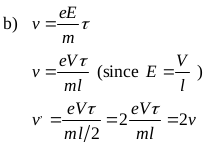# Qn) When potential difference is applied between the ends of conductor the electrons will flow through the conductor with a constant average velocity. a) Name the velocity b) A potential ‘V’is applied across a conductor of length ‘l’. Keeping the potential difference same, the length of the conductor is halved. How does the velocity of electrons change? c) Write the colour code of the resistor of resistance (540±10%) MΩ

a) Drift velocityThe drift velocity becomes twice the initial velocity.

c)The drift velocity becomes twice the initial velocity.